# Justify that the following reactions are redox reactions:

Question:

Justify that the following reactions are redox reactions:

(a) $\mathrm{CuO}(\mathrm{s})+\mathrm{H}_{2}(\mathrm{~g}) \rightarrow \mathrm{Cu}(\mathrm{s})+\mathrm{H}_{2} \mathrm{O}(\mathrm{g})$

(b) $\mathrm{Fe}_{2} \mathrm{O}_{3}(\mathrm{~s})+3 \mathrm{CO}(\mathrm{g}) \rightarrow 2 \mathrm{Fe}(\mathrm{s})+3 \mathrm{CO}_{2}(\mathrm{~g})$

(c) $4 \mathrm{BCl}_{3}(\mathrm{~g})+3 \mathrm{LiAlH}_{4}(\mathrm{~s}) \rightarrow 2 \mathrm{~B}_{2} \mathrm{H}_{6}(\mathrm{~g})+3 \mathrm{LiCl}(\mathrm{s})+3 \mathrm{AlCl}_{3}(\mathrm{~s})$

(d) $2 \mathrm{~K}(\mathrm{~s})+\mathrm{F}_{2}(\mathrm{~g}) \rightarrow 2 \mathrm{~K}+\mathrm{F}-(\mathrm{s})$

(e) $4 \mathrm{NH}_{3}(\mathrm{~g})+5 \mathrm{O}_{2}(\mathrm{~g}) \rightarrow 4 \mathrm{NO}(\mathrm{g})+6 \mathrm{H}_{2} \mathrm{O}(\mathrm{g})$

Solution:

(a) $\mathrm{CuO}_{(s)}+\mathrm{H}_{2(g)} \longrightarrow \mathrm{Cu}_{(s)}+\mathrm{H}_{2} \mathrm{O}_{(g)}$

Let us write the oxidation number of each element involved in the given reaction as: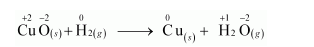Here, the oxidation number of $\mathrm{Cu}$ decreases from $+2$ in $\mathrm{CuO}$ to 0 in $\mathrm{Cu}$ i.e., $\mathrm{CuO}$ is reduced to $\mathrm{Cu}$. Also, the oxidation number of $\mathrm{H}$ increases from 0 in $\mathrm{H}_{2}$ to $+1$ in $\mathrm{H}_{2} \mathrm{O}$ i.e., $\mathrm{H}_{2}$ is oxidized to $\mathrm{H}_{2} \mathrm{O}$. Hence, this reaction is a redox reaction.

(b) $\mathrm{Fe}_{2} \mathrm{O}_{3(s)}+3 \mathrm{CO}_{(g)} \longrightarrow 2 \mathrm{Fe}_{(s)}+3 \mathrm{CO}_{2(g)}$

Let us write the oxidation number of each element in the given reaction as: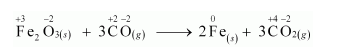Here, the oxidation number of $\mathrm{Fe}$ decreases from $+3$ in $\mathrm{Fe}_{2} \mathrm{O}_{3}$ to 0 in $\mathrm{Fe} \mathrm{i} . \mathrm{e} ., \mathrm{Fe}_{2} \mathrm{O}_{3}$ is reduced to $\mathrm{Fe}$. On the other hand, the oxidation number of $\mathrm{C}$ increases from $+2$ in $\mathrm{CO}$ to $+4$ in $\mathrm{CO}_{2}$ i.e., $\mathrm{CO}$ is oxidized to $\mathrm{CO}_{2}$. Hence, the given reaction is a redox reaction.

(c) $4 \mathrm{BCl}_{3(g)}+3 \mathrm{LiAlH}_{4(s)} \longrightarrow 2 \mathrm{~B}_{2} \mathrm{H}_{6(g)}+3 \mathrm{LiCl}_{(s)}+3 \mathrm{AlCl}_{3(s)}$

The oxidation number of each element in the given reaction can be represented as: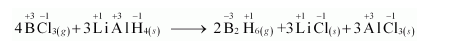In this reaction, the oxidation number of $\mathrm{B}$ decreases from $+3$ in $\mathrm{BCl}_{3}$ to $-3$ in $\mathrm{B}_{2} \mathrm{H}_{6}$. i.e., $\mathrm{BCl}_{3}$ is reduced to $\mathrm{B}_{2} \mathrm{H}_{6}$. Also, the oxidation number of $\mathrm{H}$ increases from $-1$ in $\mathrm{LiAlH}_{4}$ to $+1$ in $\mathrm{B}_{2} \mathrm{H}_{6}$ i.e., LiAlH $_{4}$ is oxidized to $\mathrm{B}_{2} \mathrm{H}_{6}$. Hence, the given reaction is a redox reaction.

(d) $2 \mathrm{~K}_{(s)}+\mathrm{F}_{2(g)} \longrightarrow 2 \mathrm{~K}^{+} \mathrm{F}^{-}(s)$

The oxidation number of each element in the given reaction can be represented as: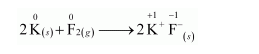In this reaction, the oxidation number of $K$ increases from 0 in $K$ to $+1$ in $K F$ i.e., $K$ is oxidized to $K F$. On the other hand, the oxidation number of $F$ decreases from 0 in $F_{2}$ to $-1$ in $\mathrm{KF}$ i.e., $\mathrm{F}_{2}$ is reduced to $\mathrm{KF}$.

Hence, the above reaction is a redox reaction.

(e) $4 \mathrm{NH}_{3(g)}+5 \mathrm{O}_{2(g)} \longrightarrow 4 \mathrm{NO}_{(g)}+6 \mathrm{H}_{2} \mathrm{O}_{(g)}$

The oxidation number of each element in the given reaction can be represented as: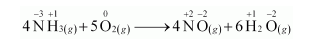Here, the oxidation number of $\mathrm{N}$ increases from $-3$ in $\mathrm{NH}_{3}$ to $+2$ in $\mathrm{NO}$. On the other hand, the oxidation number of $\mathrm{O}_{2}$ decreases from 0 in $\mathrm{O}_{2}$ to $-2$ in $\mathrm{NO}$ and $\mathrm{H}_{2} \mathrm{O}$ i.e., $\mathrm{O}_{2}$ is reduced. Hence, the given reaction is a redox reaction.